Courses

Test: Physical World- 1

10 Questions MCQ Test Physics For JEE | Test: Physical World- 1

Description
Attempt Test: Physical World- 1 | 10 questions in 10 minutes | Mock test for JEE preparation | Free important questions MCQ to study Physics For JEE for JEE Exam | Download free PDF with solutions
QUESTION: 1

Solution:
• Marie Curie became the first woman to be awarded the Nobel prize and the first person to obtain two Nobel prizes when she won the prize for the discovery of Polonium and Radium in 1911.
• Though it was Henri Becquerel that discovered radioactivity, it was Marie Curie who coined the term.
• The radioactivity of uranium was discovered in 1896 by Henri Becquerel.
QUESTION: 2

Which is the strongest fundamental force of nature?

Solution:

The strong nuclear force, also called the strong nuclear interaction, is the strongest of the four fundamental forces of nature.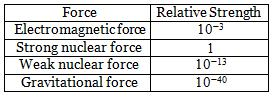QUESTION: 3

Which force operates among the heavier elementary particles?

Solution:
• The strong interaction is the attractive force that brings quarks (elementary particles) together to form nucleons (protons and neutrons).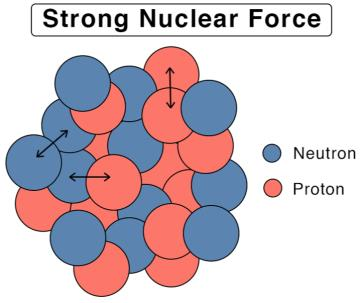• Strong nuclear force operates among the heavier elementary particles and the nucleons.
QUESTION: 4

Electromagnetic force is stronger than gravitational force by a factor of:

Solution:

The electromagnetic force is stronger than gravitational force by a factor of 1036

QUESTION: 5

Solution:
• Jagadish Chandra Bose discovered the ultra-short waves. He attended Cambridge after studying physics at Calcutta University.
• He was the first to demonstrate radio communication with millimeter wavelengths, which fall in the 30GHz to 300GHz spectrum.
QUESTION: 6

The wave nature of electrons is used in which of the following devices?

Solution:
• The electron microscope uses the wave nature of electrons for its functioning.
• It uses a beam of accelerated electrons as a source of illumination.

Electron Microscope: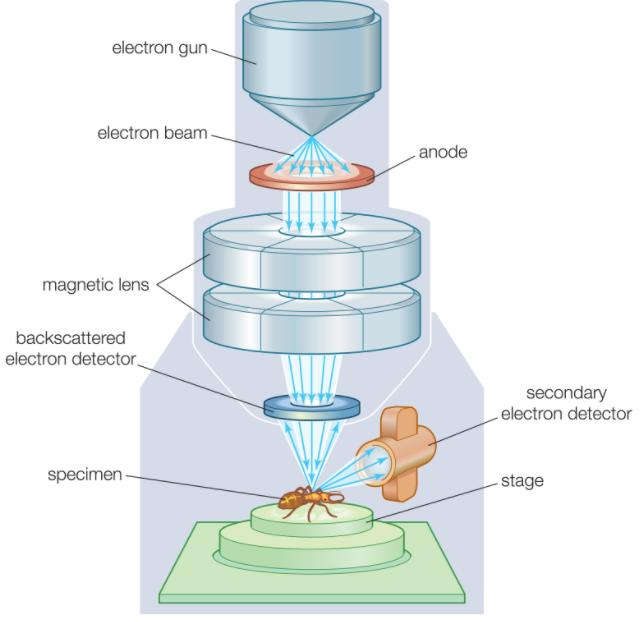QUESTION: 7

According to Einstein’s theory, mass and energy are related as:

Solution:
• Einstein's equation E = mc2 shows that energy and mass are interchangeable.
• The theory of special relativity explains how space and time are linked for objects that are moving at a consistent speed in a straight line.
• One of its most famous aspects concerns objects moving at the speed of light.
QUESTION: 8

On which laws, is the principle of rocket propulsion based?

Solution:
• Propulsion is the act of moving or pushing an object forward. A propulsion system is an engine that produces thrust to push an object, such as an airplane or rocket, forward.
• The propulsion of all rockets is explained by the same physical principle: Newton’s third law of motion.
• It states that all forces exist in pairs: if one object A exerts a force FA on a second object B, then B simultaneously exerts a force FB on A, and the two forces are equal and opposite: FA = −FB.
• A rocket’s acceleration depends on three major factors: the exhaust velocity, the rate the exhaust is ejected, and the mass of the rocket.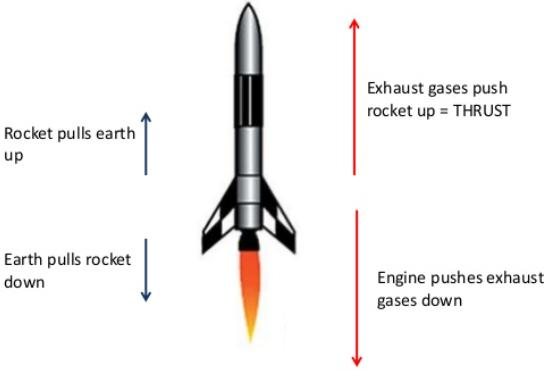• To achieve the high speeds needed to hop continents, obtain orbit, or escape Earth’s gravity altogether, the mass of the rocket other than fuel must be as small as possible.
QUESTION: 9

The phenomenon of Photoelectric effect is used in:

Solution:
• A photocell is a practical application of the phenomenon of the photoelectric effect
• When the light of frequency greater than the threshold frequency of the cathode material falls on the cathode, photoelectrons are emitted.
• These electrons are collected by the anode and an electric current starts flowing in the external circuit.
• The current increases with the increase in the intensity of light. The current would stop, if the light does not fall on the cathode.
QUESTION: 10

Scientific method involves

Solution:

Systematic observations, controlled experiments, qualitative and quantitative reasoning, mathematical modelling, prediction and verification.Use Code STAYHOME200 and get INR 200 additional OFF Use Coupon Code

Track your progress, build streaks, highlight & save important lessons and more!

Similar Content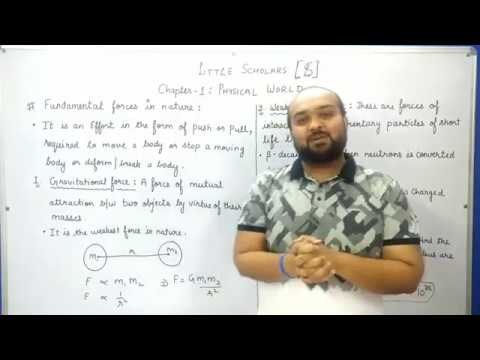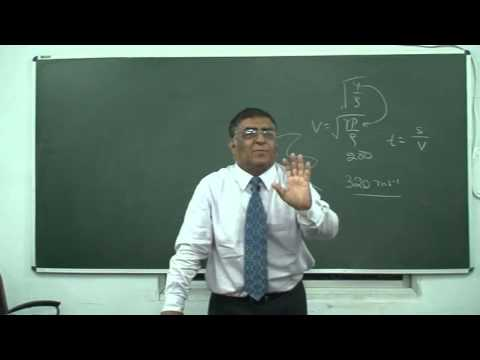Related tests